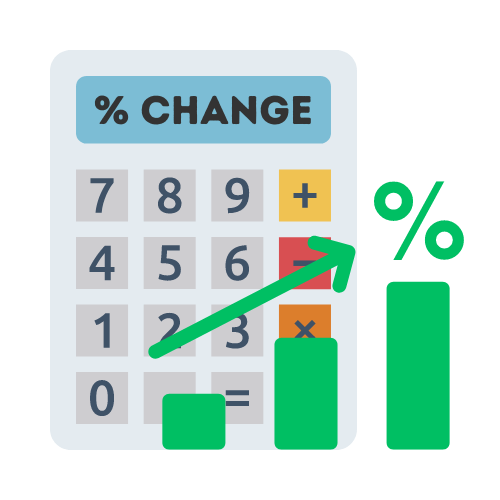# Percentage Change Calculator

## Calculate percentage change easily with the percent change calculator

The percent change is:

## What is percentage change?

Percentage change is the most common way for measuring change between two numbers. It can be represented as a positive number or negative number, depending on whether the second value has increased or decreased.

## How to calculate percentage change?

The easiest way to calculate percentage change is to use a percentage change calculator like the one on this page. However, it's also good to know how to do things by yourself when necessary. So, how do you calculate percentage change manually?

Doing the calculation manually is fairly easy. First you need to take note of the two numbers that you want to compare.

You begin the calculation by taking the second value, and divide it by the original value, then multiply the remainder by 100.

## Why do we calculate percentage change?

Percentage change is used to compare changes in two numbers. It allows us to answer questions such as: How much did sales increase this month? How much has budget been cut from last year? What is the percentage growth of my social media following over the last month, quarter or year?

## When to use percentage change?

Percentage change is used to compare results between two different periods or between two different sets of data, and it measures the difference in percentages.

For instance, when you compare the price of two houses of the same type in two different years, you can use percentage change to present the difference between the two sale prices for that duration as a percentage.

## What is the formula for percentage change?

The formula for percentage change is:

`Percent increase (%) = (New Value - Original Value) / Original Value`

This percent change formula gives you the total percentage difference between two values. When calculating the percentage change, you start by subtracting the original value from the new value. This gives you a decimal value as the result, then multiply it by 100 to convert it to a percentage.